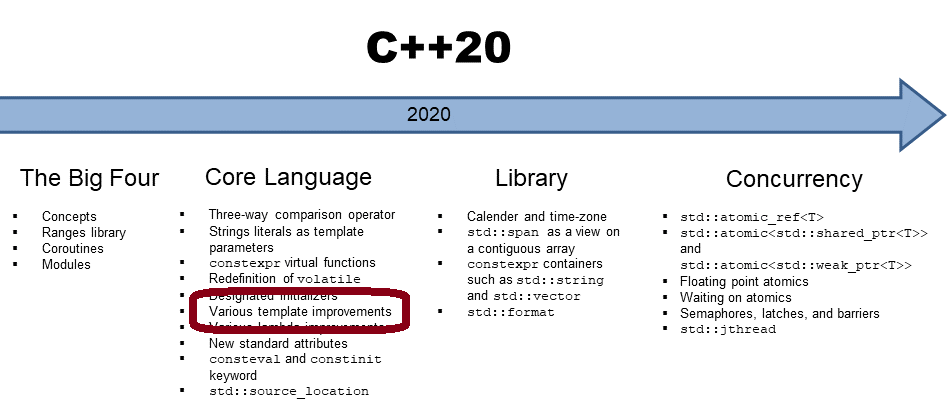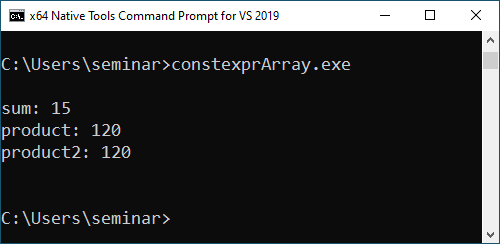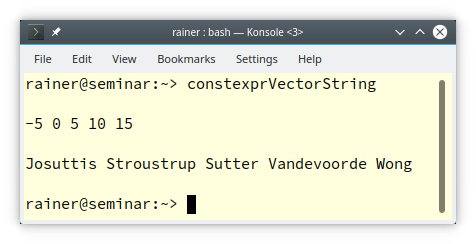# constexpr std::vector and std::string in C++20

Probably the most viral keyword in modern C++ is `constexpr`. With C++20, we have a` constexpr std::vector` and a` constexpr std::string`. Additionally, both containers can be manipulated with the `constexpr` algorithms of the Standard Template Library.In this post, I want to calculate a few numbers’ sum and product at compile time. Depending on the applied C++ standard, this is challenging or quite comfortable to achieve. Let’s start with C++11.

A variadic template is a template that can be invoked with an arbitrary number of arguments. By using the ellipse (…)` tails` becomes a so-called parameter pack. Parameter packs can only be packed and unpacked. If the ellipse is left from `tails`, the parameter pack is packed. If the ellipse is right from tail`s`, the parameter pack is unpacked.

```// compiletimeVariadicTemplates.cpp

#include <iostream>

template<int...>
struct sum;

template<>
struct sum<> {
static constexpr int value = 0;
};

template<int i, int... tail>
struct sum<i, tail...> {
static constexpr int value = i + sum<tail...>::value;
};

template<int...>                                  // (1)
struct product;

template<>                                        // (2)
struct product<> {
static constexpr int value = 1;
};

template<int i, int... tail>                      // (3)
struct product<i, tail...> {
static constexpr int value = i * product<tail...>::value;
};

int main() {

std::cout << std::endl;

std::cout << "sum<1, 2, 3, 4, 5>::value: " << sum<1, 2, 3, 4, 5>::value << std::endl;
std::cout << "product<1, 2, 3, 4, 5>::value: " << product<1, 2, 3, 4, 5>::value << std::endl;

std::cout << std::endl;

}
```

##Modernes C++ Mentoring

Be part of my mentoring programs:

• "Fundamentals for C++ Professionals" (open)
• "Design Patterns and Architectural Patterns with C++" (open)
• "C++20: Get the Details" (reopens December 2023)
• Do you want to stay informed about my mentoring programs: Subscribe via E-Mail.

The program calculates the sum and the product of the numbers 1 to 5 at compile time. In the case of the function template `product`, line (1) declares the primary template, line (2) the full specialization for zero arguments, and line (3) the partial specialization for at least one argument. The definition of the primary template (1) is unnecessary if you don’t use it. The partial specialization (3) starts a recursive instantiation, which ends when all template arguments are consumed. In this case, the full specialization for zero arguments kicks in as the boundary condition. To know how this pack expansion is performed, study the example compileTimeVariadicTemplates.cpp at C++ Insights.Thanks to fold expression, calculating at compile-time becomes way more manageable.

## Fold Expressions in C++17

With C++17, we can directly reduce a parameter pack with a binary operator.

```// compiletimeFoldExpressions.cpp

#include <iostream>

template<typename... Args>
auto sum(const Args&... args)
{
return (args + ...);
}

template<typename... Args>
auto product(const Args&... args)
{
return (args * ...);
}

int main() {

std::cout << std::endl;

std::cout << "sum(1, 2, 3, 4, 5): " << sum(1, 2, 3, 4, 5) << std::endl;
std::cout << "product(1, 2, 3, 4, 5): " << product(1, 2, 3, 4, 5) << std::endl;

std::cout << std::endl;

}
```

The program `compiletimeFoldExpressions.cpp` produces the same result at compile-time, such as the previous program compileTimeVariadicTemplates.cpp.Of course, there is more to write about fold expression. Please read the details in my previous post to Fold Expressions.

Now I want to dive into C++20.

## constexpr Containers and Algorithms in C++20

C++20 supports the `constexpr` containers` std::vector` and `std::string. constexpr` means, in this case, that the member functions of both containers can be applied at compile-time.

Before I write about both containers, I have to make a short detour to C++17. The reason is simple: No compiler so far supports the `constexpr` `std::vector` and` std::string`. In contrast, the GCC and the Microsoft Compiler support the `constexpr` algorithms of the STL.

In my following example, I use instead of a `constexpr std::vector` a `constexpr std::array. `Since C++17` std::array` can be declared as `constexpr: constexpr std::array myArray{1, 2, 3}. `

Now starts the fun part. With C++20, you can use a` std::array` at compile-time.

```// constexprArray.cpp

#include <iostream>
#include <numeric>
#include <array>

int main() {

std::cout << std::endl;

constexpr std::array myArray{1, 2, 3, 4, 5};                                     // (1)
constexpr auto sum = std::accumulate(myArray.begin(), myArray.end(), 0);         // (2)
std::cout << "sum: "  << sum << std::endl;

constexpr auto product = std::accumulate(myArray.begin(), myArray.end(), 1,      // (3)                                             std::multiplies<int>());
std::cout << "product: "  << product << std::endl;

constexpr auto product2 = std::accumulate(myArray.begin(), myArray.end(), 1,     // (4)                                              [](auto a, auto b) { return a * b;});
std::cout << "product2: "  << product2 << std::endl;

std::cout << std::endl;

}
```

The` std::array` (1) and all results of the calculations are declared as `constexpr`. Line (2) calculates the sum of all elements, and lines (3) and (4) calculate the product of all elements of` myArray`. Line 2 is valid because `myArray` is a `constexpr` container and the algorithm `std::accumulate` is declared as `constexpr`. Lines (3) and (4) are more interesting. The call operator  `std::multiplies` is `constexpr` and since C++17 lambda expression can be `constexpr`.

Here is the output of the program:Thanks to the Compiler Explorer, I can present the results way more impressive. Here are the relevant assembler instructions of GCC.Lines 19, 29, and 39 show that the results of the array calculations become values in the assembler instructions.  This means `std::accumulate` is performed at compile-time, and the calculation result is just available at run-time.

As I already mentioned, no compiler so far supports a `constexpr std::vector` or a` constexpr std::string`. Consequently, I must cheat and assume that my compiler fully supports both `constexpr` containers.

```// constexprVectorString.cpp

#include <algorithm>
#include <iostream>
#include <string>
#include <vector>

int main() {

std::cout << std::endl;

constexpr std::vector myVec {15, -5, 0, 5, 10};
constexpr std::sort(myVec.begin(), myVec.end());
for (auto v: myVec) std::cout << v << " ";
std::cout << "\n\n";

using namespace std::string_literals;
constexpr std::vector<std::string> myStringVec{"Stroustrup"s, "Vandevoorde"s,                                                    "Sutter"s, "Josuttis"s, "Wong"s };
constexpr std::sort(myStringVec.begin(), myStringVec.end());
for (auto s: myStringVec) std::cout << s << " ";

std::cout << "\n\n";

}
```

With C++20, you can sort a `std::vector` or a `std::string` at compile-time.## What’s next?

C++20 adds many convenience functions to make working with containers of the Standard Template Library easier. For example, due to the functions std::erase and std::erase_if, the deletion of container elements works like a charm. When you want to know if a specific element is in an associative container, the member function `contains` is quite handy.

Thanks a lot to my Patreon Supporters: Matt Braun, Roman Postanciuc, Tobias Zindl, G Prvulovic, Reinhold Dröge, Abernitzke, Frank Grimm, Sakib, Broeserl, António Pina, Sergey Agafyin, Андрей Бурмистров, Jake, GS, Lawton Shoemake, Jozo Leko, John Breland, Venkat Nandam, Jose Francisco, Douglas Tinkham, Kuchlong Kuchlong, Robert Blanch, Truels Wissneth, Kris Kafka, Mario Luoni, Friedrich Huber, lennonli, Pramod Tikare Muralidhara, Peter Ware, Daniel Hufschläger, Alessandro Pezzato, Bob Perry, Satish Vangipuram, Andi Ireland, Richard Ohnemus, Michael Dunsky, Leo Goodstadt, John Wiederhirn, Yacob Cohen-Arazi, Florian Tischler, Robin Furness, Michael Young, Holger Detering, Bernd Mühlhaus, Matthieu Bolt, Stephen Kelley, Kyle Dean, Tusar Palauri, Dmitry Farberov, Juan Dent, George Liao, Daniel Ceperley, Jon T Hess, Stephen Totten, Wolfgang Fütterer, Matthias Grün, Phillip Diekmann, Ben Atakora, Ann Shatoff, Rob North, Bhavith C Achar, and Marco Parri Empoli.

Thanks, in particular, to Jon Hess, Lakshman, Christian Wittenhorst, Sherhy Pyton, Dendi Suhubdy, Sudhakar Belagurusamy, Richard Sargeant, Rusty Fleming, John Nebel, Mipko, Alicja Kaminska, Slavko Radman, and David Poole.

 My special thanks to EmbarcaderoMy special thanks to PVS-StudioMy special thanks to Tipi.buildMy special thanks to Take Up Code## Seminars

I’m happy to give online seminars or face-to-face seminars worldwide. Please call me if you have any questions.

### Standard Seminars (English/German)

Here is a compilation of my standard seminars. These seminars are only meant to give you a first orientation.

• C++ – The Core Language
• C++ – The Standard Library
• C++ – Compact
• C++11 and C++14
• Concurrency with Modern C++
• Design Pattern and Architectural Pattern with C++
• Embedded Programming with Modern C++
• Generic Programming (Templates) with C++

#### New

• Clean Code with Modern C++
• C++20

### Contact Me

Modernes C++ Mentoring,Tags:
0 replies